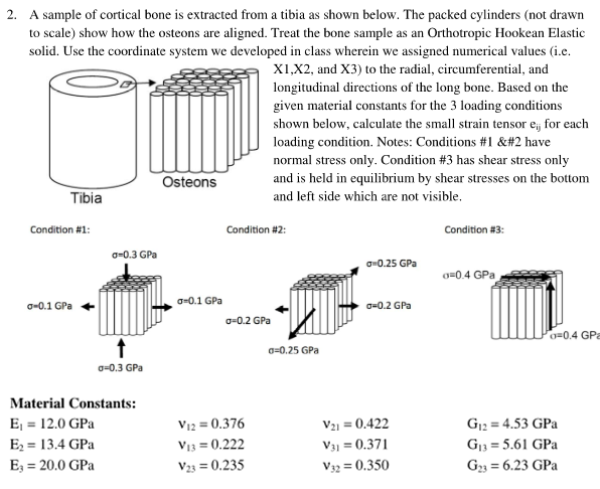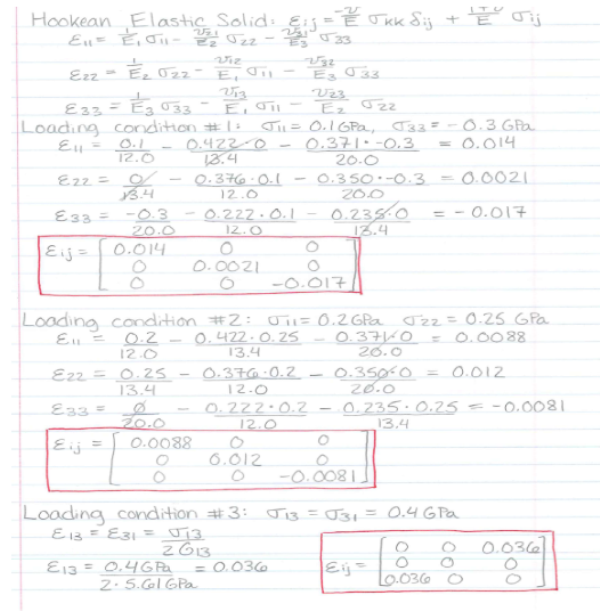A sample of cortical bone is extracted from a tibia as shown below. The packed cylinders show how the osteons are aligned. Treat the bone sample as an orthotropic hookean elastic solid. Use the coordinate system we developed in class wherein we assigned numerical values the the radial, circumferential, and longitudinal directions of the long bone. Based on the given material constants for the 3 loading conditions shown below, calculate the small strain tensor for each loading condition.A sample of cortical bone is extracted from a tibia as shown below. The packed cylinders show how the osteons are aligned. Treat the bone sample as an orthotropic hookean elastic solid. Use the coordinate system we developed in class wherein we assigned numerical values the the radial, circumferential, and longitudinal directions of the long bone. Based on the given material constants for the 3 loading conditions shown below, calculate the small strain tensor for each loading condition.

biomechanics page 1 biomechanics biomechanics biomechanics biomechanics biomechanics biomechanics biomechanics biomechanics page 2